# 说说的那道求面积的小学六年级几何题

zhejiang wenzhou skinshoe wet，rain flooding water will not fat！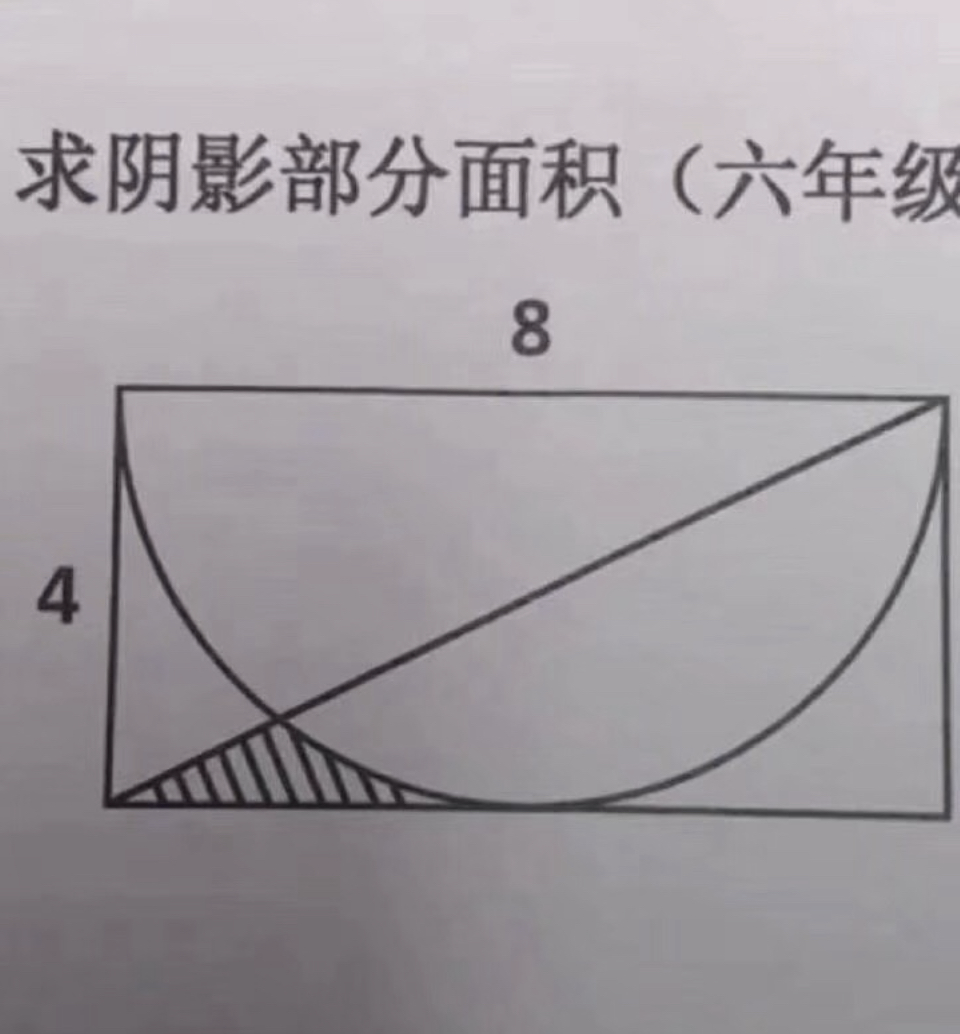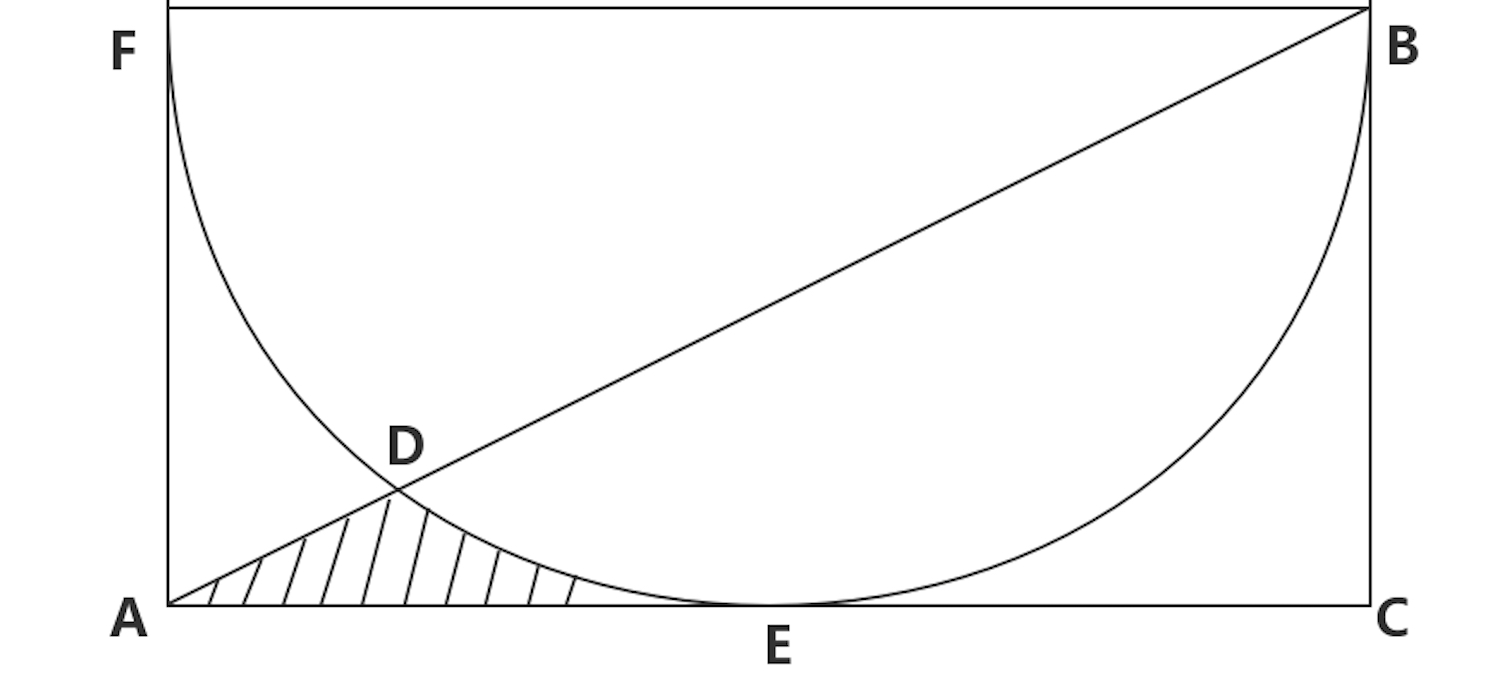$S_{ADE}=S_{\triangle_{ABC}}-S_{BCE}-S_{DBE}$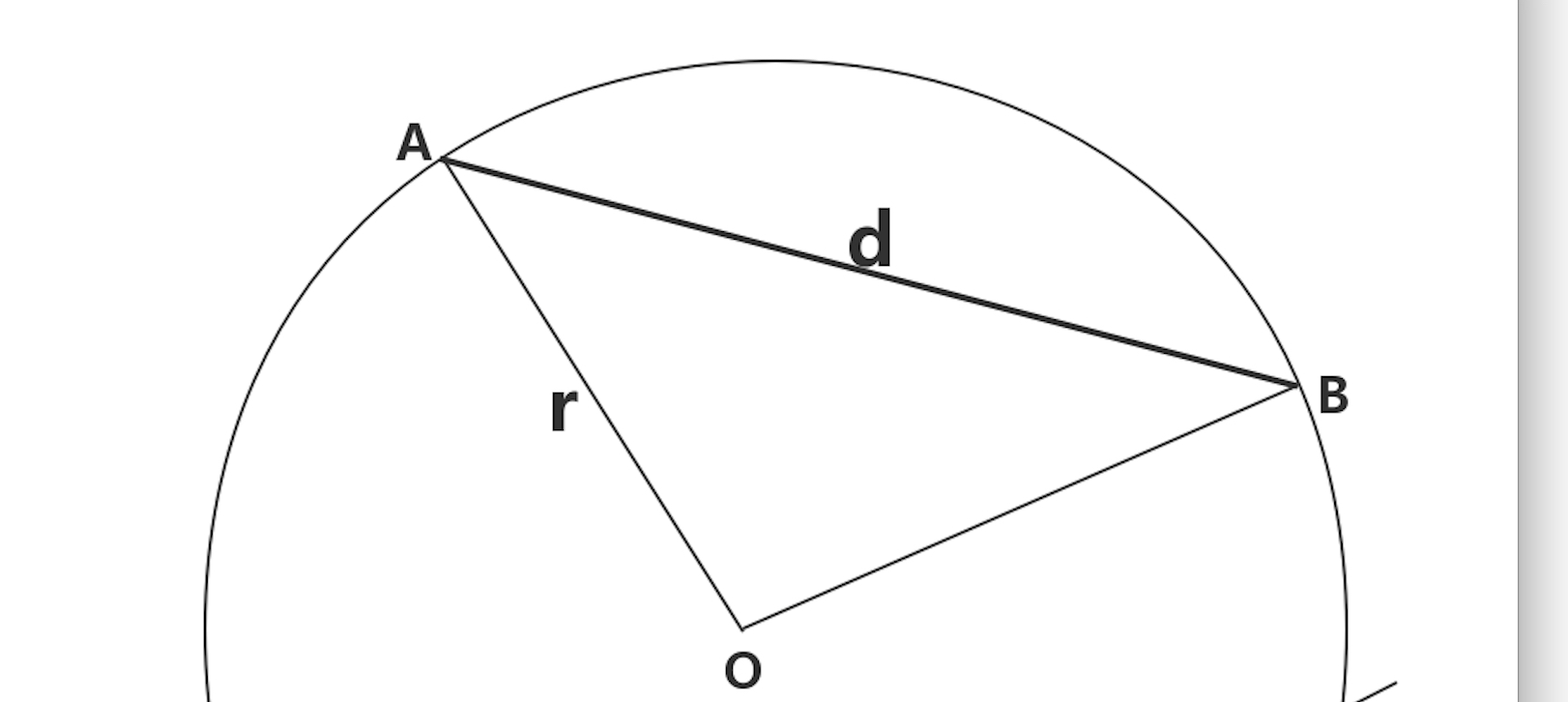$\theta=2\times \arcsin(\dfrac{d}{2r})$

$S_{扇_{AOB}}=\dfrac{\theta}{360}\times\pi r^2$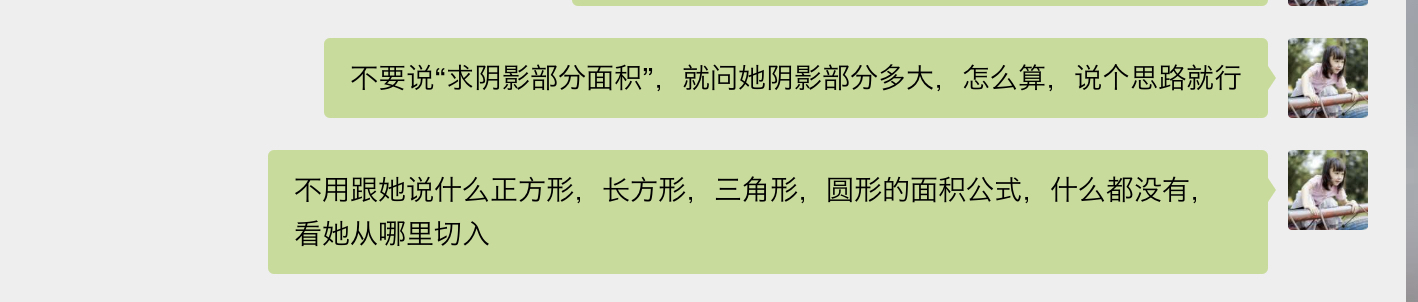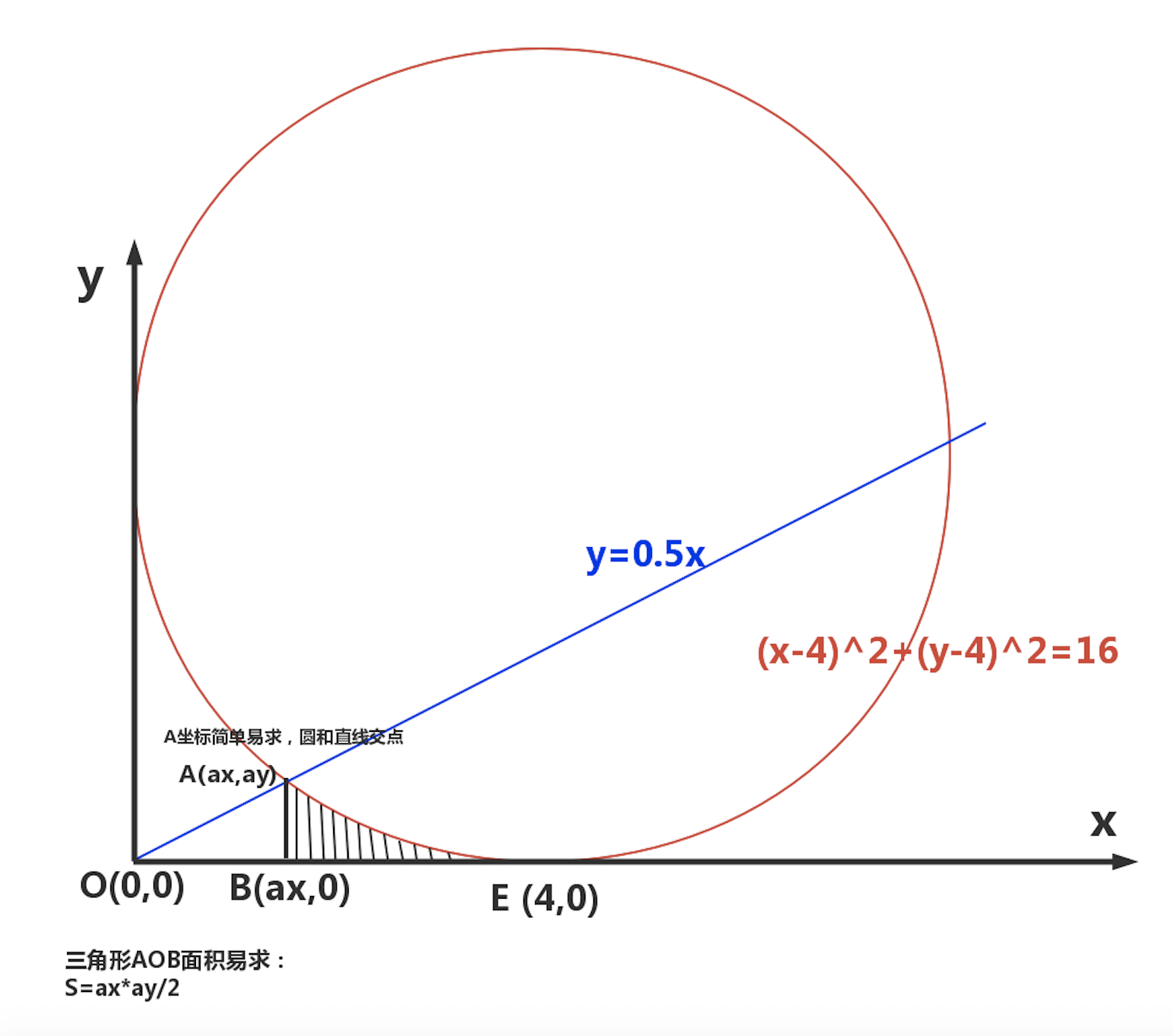$y=\sqrt {16-(x-4)^2}+4$

$S_{ABE}=\displaystyle \int_{a_x}^{4} (\sqrt {16-(x-4)^2}+4)dx$

$S_{原题}=\dfrac{a_x\times a_y}{2}+\displaystyle \int_{a_x}^{4} (\sqrt {16-(x-4)^2}+4)dx$

$y=\sqrt {16-(x-4)^2}+4=\dfrac{x}{2}$

• 对经验的总结和自省能力；
• 抽象的逻辑推理能力；

for 长方形范围内的每一个像素p:
if p 属于 \$阴影部分   # 可以基于像素的三原色来判断
count ++
endif
endfor